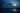# Cold v Hot Observables

Angular: The Full Gamut EditionCharlie Greenman
September 06, 2020One of the foundational concepts of RxJS is understanding what the difference between cold and hot observables is. At its core this difference is:

There is a lot to unpack here, so let's dissect.

## Cold Observables

To re-iterate, an observable is called `cold`, when the data is produced inside of the observable. An observable is only instantiated, once the `subscribe` is called. So, we can have something like this:

``import { Subject } from 'rxjs';const randomVal\$ = new Subject().next(Math.random());}``

That will actually create an observable, but it will not be called until we add a `subscribe` to the code.

``````import { Subject } from 'rxjs';const randomVals\$ = new Subject().next(Math.random());// Subscribe to run the combined functions
randomVals\$.subscribe(x => console.log(x));``````

For that reason, it's possible to have it subscribe mutliple times to a singular observable, yet get different results each time. If in the above code, we created two subscribes, so that our code now looks like this:

``````import { Subject } from 'rxjs';const randomVals\$ = new Subject().next(Math.random());// Subscribe to run the combined functions
randomVals\$.subscribe(x => console.log(x));// 0.8771441274333971 (random number)
randomVals\$.subscribe(x => console.log(x));// 0.09728173083079916 (random number)``````

As we can see in the comments above, each subscribe will give us a new value.

### Maintaining Same Value for Both Subscribers - Venture into the Land of Hot

However, let's say that we wanted to maintain the same random value for both subscribers. What we would do, is create a constant outside of the subject logic, so that it remains the same value for both.

``````import { Subject } from 'rxjs';const random = Math.random();const randomVal\$ = new Subject().next(random);// Subscribe to run the combined functions
randomVals\$.subscribe(x => console.log(x));// 0.8771441274333971 (random number)
randomVals\$.subscribe(x => console.log(x));// 0.8771441274333971 (random number)``````

Here, we have moved the data producer(i.e. `Math.random()`) out of observable. So, to go back to our definition of a hot, vs cold observable. Being that this data was produced outside of the observable, our observable now just went from being a cold observable to a hot observable. However, as time goes on, you will have to apply this (hot v cold) logic to every scenario to truly understand where the data is being produced.

## Hot Observables

So, while we have produced a good example for what a cold vs hot observable is, we have not produced an enterprise example, that really drives it home. The truth is, that actually `@ngrx/store` is based on hot observables. In particular when using `@ngrx/effects`.

``````@Effect() loadPosts\$ = this.dataPersistence.fetch(

As we can see, we are passing in an action that already exists. In fact, we are using nrwl's utility function `dataPersistence` to make sure our data persists. If we dig deep, we will see that it extends this core functionality of `ngrx/effects`:

``````/**
* @whatItDoes Provides convenience methods for implementing common operations of persisting data.
*/export declare class DataPersistence<T> {
store: Store<T>;
actions: Actions;constructor(store: Store<T>, actions: Actions);``````
``export declare class Actions<V = Action> extends Observable<V> {constructor(source?: Observable<V>);lift<R>(operator: Operator<V, R>): Observable<R>;//..}``

The actions are extending the Observable type annotation. This is immediately apparent if using the ngrx/effects 's createEffect method. However, on principal, the Angular: The Full Gamut series uses enterprise examples, even when trying to explain core concepts

We are accepting data from an action, turn all actions within our app as an observable, thereby making the data produced outside of our effects. This is a classic scenario that happens time and time again, that is in truth a hot observable. Knowing this truth is very valuable, especially when it comes to unit testing your code.

## Why Make an Observable Hot?

To re-iterate, an Observable by default is cold. So, why would one want to make an observable hot? This has value in two particular situations:

1. Have multiple subscribers get the same data

2. If you would have to create a new value time and time again within your observables subscribe(websocket, action etc.).

3. Separation of concern. Within \@ngrx/store we have files, and pieces of our state management that do different things. By creating a hot observable, it allows us to pass through the data, use a hot observable, and keep with separation of concerns.